# SSAT Middle Level Math : Tables

## Example Questions

1 3 Next →

### Example Question #1 : Make A Line Plot To Display A Data Set Of Measurements In Fractions And Solve Problems: Ccss.Math.Content.5.Md.B.2

Mrs. Smith’s class made a line plot to show the amount of sugar that each student consumes in a given day.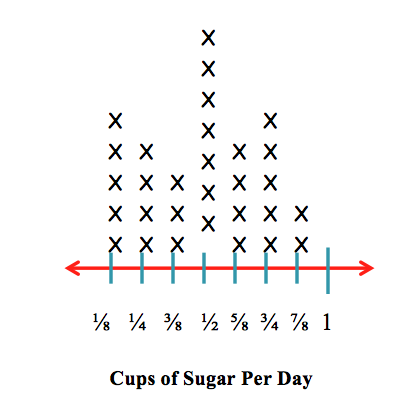How many students consumecup of sugar?Explanation:

Each X represents one person. There areXs above thetick mark on the line plot.

### Example Question #1 : Make A Line Plot To Display A Data Set Of Measurements In Fractions And Solve Problems: Ccss.Math.Content.5.Md.B.2

Mrs. Smith’s class made a line plot to show the amount of sugar that each student consumes in a given day.How many students consumecup of sugar?Explanation:

Each X represents one person. There areXs above thetick mark on the line plot.

### Example Question #2 : Make A Line Plot To Display A Data Set Of Measurements In Fractions And Solve Problems: Ccss.Math.Content.5.Md.B.2

Mrs. Smith’s class made a line plot to show the amount of sugar that each student has in a given day.How many students have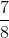cups of sugar?Explanation:

Each X represents one person. There areXs above thetick mark on the line plot.

### Example Question #41 : Measurement & Data

Mrs. Smith’s class made a line plot to show the amount of sugar that each student has in a given day.How many students haveorcups of sugar?Explanation:

To solve this problem, we have to add the number of Xs above thetick mark to the number of Xs above thetick mark.

There areXs above thetick mark andXs above thetick mark.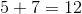### Example Question #21 : How To Find The Answer From A Table

Mrs. Smith’s class made a line plot to show the amount of sugar that each student has in a given day.How many students have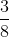of a cup of sugar?Explanation:

Each X represents one person. There areXs above thetick mark on the line plot.

### Example Question #41 : Measurement & Data

Mrs. Smith’s class made a line plot to show the amount of sugar that each student has in a given day.How many students have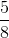of a cup of sugar?Explanation:

Each X represents one person. There areXs above thetick mark on the line plot.

### Example Question #21 : How To Find The Answer From A Table

Find: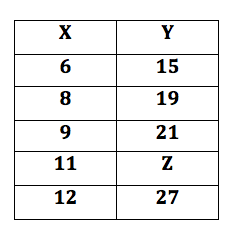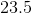Explanation:

If you are faced with a chart and a missing number, you need to figure out the pattern in the pairs that are shown.

If you think you have discovered the pattern, make sure to test it on each known pair and test if it is true for every pair.

The pattern will always stay the same. For these set of numbers the formula is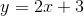.

Therefore,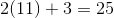.

### Example Question #631 : Ssat Middle Level Quantitative (Math)

The table below gives the number of students who scored in each of five  ranges on a standardized test.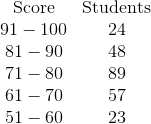No student scored below 51.

What percent of the students scored 71 or better (nearest whole number)?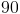%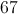%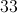%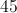%%

Explanation:

Add the numbers of students whose scores fell in the 71-80, 81-90, and 91-100 ranges: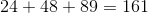Now add the number of students who took the test.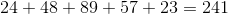To find what percent 161 is of 241, evaluate: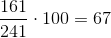67% of the students scored 71 or better.

1 3 Next →

### All SSAT Middle Level Math Resources